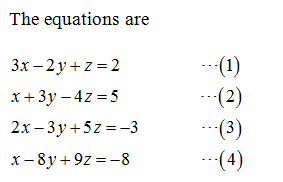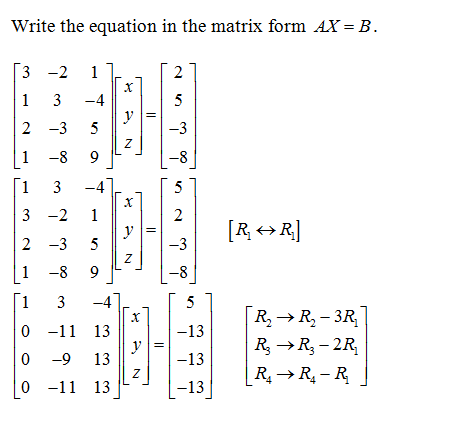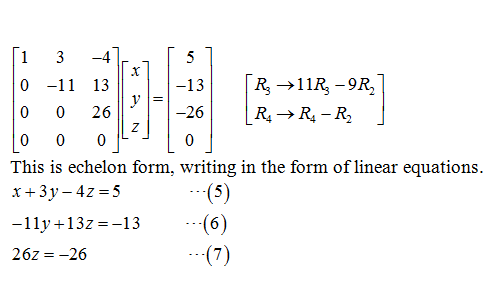# Solve the following system of equations. (Enter your answers as a comma-separated list. If there are infinitely many solutions, enter a parametric solution using t and/or s. If there is no solution, enter NONE.)3x − 2y + z = 2x + 3y − 4z = 52x − 3y + 5z = −3x − 8y + 9z = −8(x, y, z)=

Question
1 views

Solve the following system of equations. (Enter your answers as a comma-separated list. If there are infinitely many solutions, enter a parametric solution using t and/or s. If there is no solution, enter NONE.)

 3x − 2y + z = 2 x + 3y − 4z = 5 2x − 3y + 5z = −3 x − 8y + 9z = −8
(x, y, z)=
check_circle

Given:Solve the equation:On further simplification,...

### Want to see the full answer?

See Solution

#### Want to see this answer and more?

Solutions are written by subject experts who are available 24/7. Questions are typically answered within 1 hour.*

See Solution
*Response times may vary by subject and question.
Tagged in